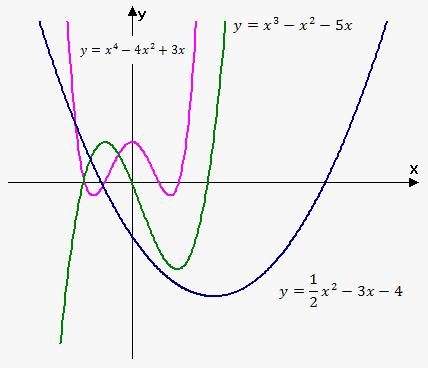# Polynomial Function

Polynomial is a function of the form ${y}={f{{\left({x}\right)}}}={a}_{{n}}{{x}}^{{n}}+{a}_{{{n}-{1}}}{{x}}^{{{n}-{1}}}+\ldots+{a}_{{2}}{{x}}^{{2}}+{a}_{{1}}{x}+{a}_{{0}}$, where n is nonnegative integer and ${a}_{{0}},\ {a}_{{1}},\ \ldots,\ {a}_{{{n}-{1}}},\ {a}_{{n}}$ are constants which are called coefficients of polynomial.

Domain of any polynomial is ${\left(-\infty,\infty\right)}$.

If ${a}_{{n}}\ne{0}$ then ${n}$ is the degree of polynomial. For example, ${f{{\left({x}\right)}}}=-{2}{{x}}^{{5}}+{3}{x}+\sqrt{{{3}}}$ is a polynomial of degree 5.

If degree of polynomial is n=1, then ${\color{blue}{{{f{{\left({x}\right)}}}={m}{x}+{b}}}}$ and it is linear function.

In case degree is 2 then ${\color{red}{{{f{{\left({x}\right)}}}={a}{{x}}^{{2}}+{b}{x}+{c}}}}$ and this function is called quadratic.

A polynomial of degree 3 ${\color{green}{{{f{{\left({x}\right)}}}={a}{{x}}^{{3}}+{b}{{x}}^{{2}}+{c}{x}+{d}}}}$ is called cubic function.

Graph of polynomial functions have different shapes. On the figure you can see graph of polynomials with degree ${n}={2},{3},{4}$.